output.to from Sideway
Draft for Information Only

# Content

```  MatLab: Scratch Pad & Arithmetic Operations   Arithmetic Operators   Matrix Arithmetic Operations    + Addition    Examples    + Unary Plus    Examples    - Subtraction    Examples    - Unary Minus    Examples    * Matrix Multiplication    Examples    / Slash or Matrix Right Division    Examples    \ Backslash or Matrix Left Division    Examples    ^ Matrix Power    Examples    ' Matrix Transpose or Complex Conjugate Transpose    Examples   Array Arithmetic Operations    + Addition    Examples    + Unary Plus    Examples    - Subtraction    Examples    - Unary Minus    Examples    .* Array Multiplication    Examples    ./ Array Right Division    Examples    .\ Array Left Division    Examples    .^ Array Power    Examples    .' Array Transpose    Examples   Arithmetic Operation Functions   Arithmetic Expressions   Operator Precedence```

## MatLab: Scratch Pad & Arithmetic Operations

Major Reference Source: MatLab Verson 7.0

Using MatLab package as a scratch pad interactively is one of the common application of the MatLab package. MatLab commands are entered at the prompt command, >>, of the Command Window through the PC keyboard. Both entered commands and evaluation results are displayed in the Command Window. All new line or lines of commands or instructions after the prompt command will be passed to MatLab for evaluation after pressing the Enter key no matter the position of mouse cursor is at the end of line or lines or not.

### Arithmetic Operators

Since all types of data in MatLab are stored in the form of arrays, MatLab provides two different kinds of arithmetic operations, i.e. array operations and matrix operations. MatLab array operations are just ordinary arithmetic operations that supports multidimenstional arrays for processing element by elemt operations. While MatLab matrix operation are ordinary matrix operations that following the rules of linear algebra. Both array operations and matrix operations share the similar symbols of operation, a period characater, "." is used to distinguish the array operations from the matrix operations. However, as the addition and subtraction for both matrix operation and array operation are the same, the period characater, "." is not necessary and the character pairs ".+" and ".-" are not used. Besides, the 1-by-1 array, scalar, is also a special type of MatLab array. A 1-by-1 array, scalar can have array operation with an array of any size also. A 1-by-1 matrix, scalar can also have martrix operation with a matrix of any size, but limited by the matrix multiplication, the 1-by-1 matrix, scalar can only be the divisor of the right and left division.

The matrix and array arithmetic includes seven types of operations:

Arithmetic
Operators
Matrix
Arithmetic Operations
Element-Wise Array
Arithmetic Operations
Syntax Description Syntax Description
+
+A Unary Plus +A Unary Plus
Subtraction
-
A-B Subtraction A-B Subtraction
-A Unary Minus -A Unary Minus
Multiplication
*
A*B Matrix
Multiplication
A.*B Array
Multiplication
Right  Division
/
A/B Forward Slash or Matrix Right Division A./B Array
Right Division
Left Division
\
A\B Backslash or Matrix Left Division A.\B Array
Left Division
Power
^
A^B Matrix Power A.^B Array Power
Transpose
'
A' Matrix Transpose or
Complex Conjugate Transpose
A.' Array Transpose

### Matrix Arithmetic Operations

The + addition operator of the expression A+B means adds matrix B to matrix A. Since only a scalar can be added by or added to a matrix of any size, unless either A or B is a scalar, A and B must have the same size.

#### Examples

• m-by-n matrix A + m-by-n matrix B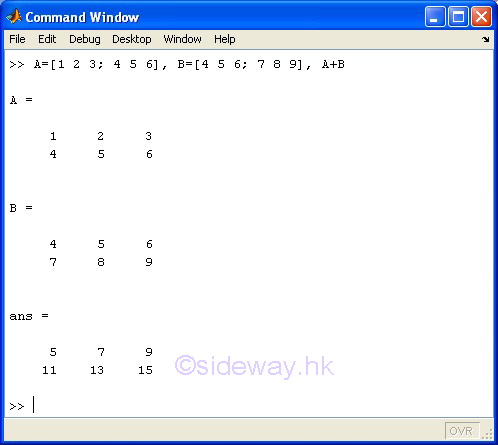• scalar S + matrix A + scalar S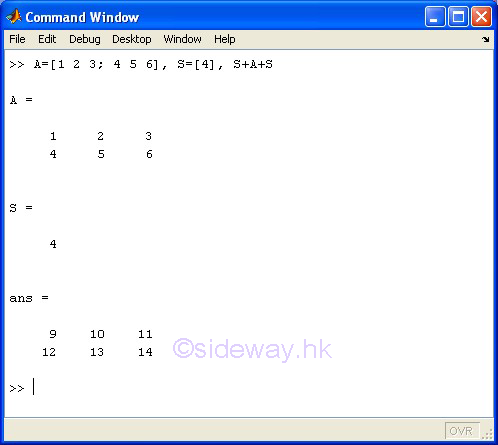#### + Unary Plus

The + unary plus operator of the expression +A means returns matrix A.

#### Examples

• +matrix A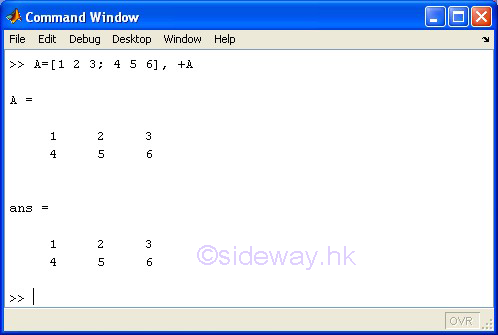#### - Subtraction

The - subtraction operator of the expression A-B means subtracts matrix B from matrix A. Since only a scalar can be subtracted by or subtracted from a matrix of any size, unless either A or B is a scalar, A and B must have the same size.

#### Examples

• m-by-n matrix A - m-by-n matrix B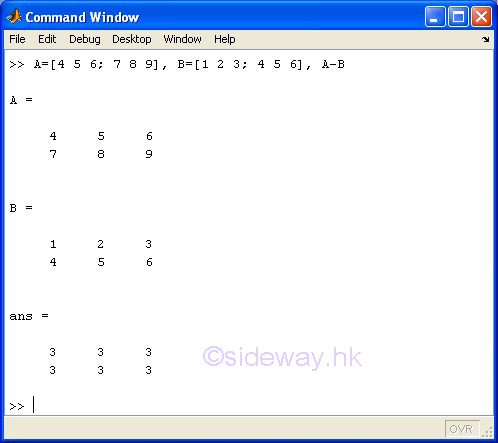• scalar S - m-by-n matrix A - scalar S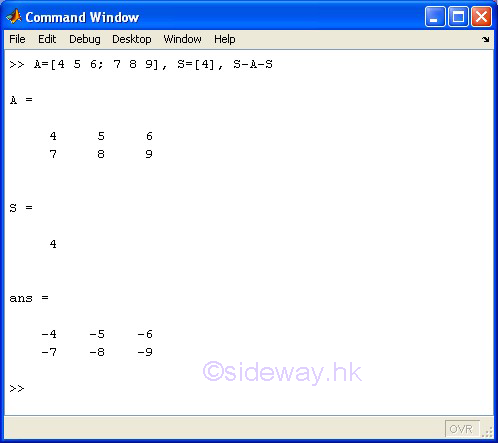#### - Unary Minus

The - unary minus operator of the expression -A means returns and negates the elements of matrix A.

#### Examples

• -matrix A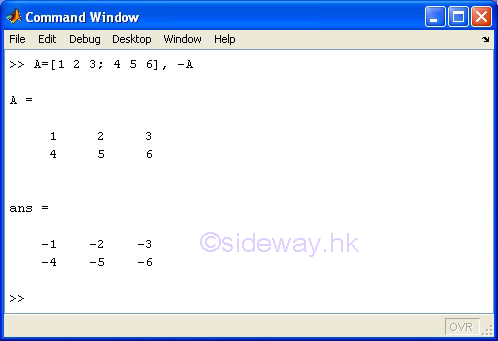#### * Matrix Multiplication

The * matrix multiplication operator of the expression A*B means to carry out the linear algebraic product of matrix A by matrix B.

Since only a scalar can be multiplicated by or multiplicated to a matrix of any size,  two or more dimensions are supported, unless either A or B is a scalar, otherwise the number of columns of matrix A must equal to the number of rows of matrix B.

#### Examples

• m-by-n matrix A * n-by-o matrix B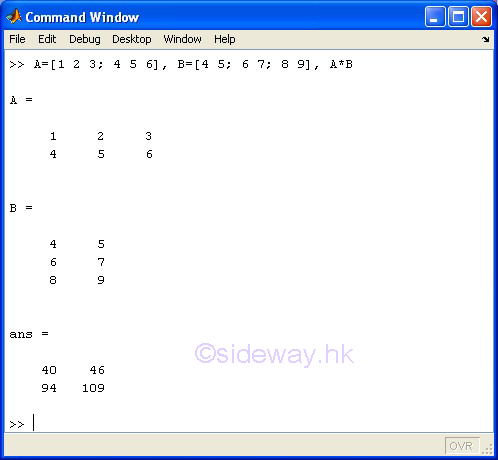• scalar S * m-by-n matrix A * scalar S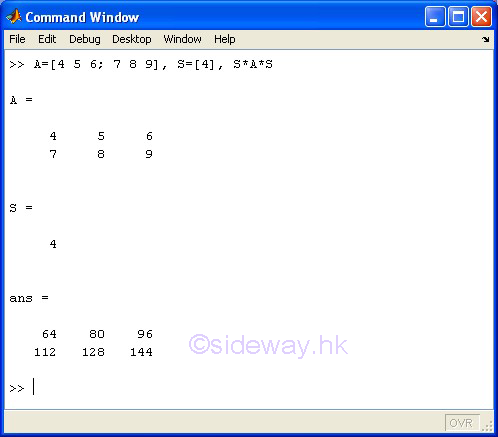#### / Slash or Matrix Right Division

The / slash or matrix right division operator of the expression A/B means to carry out the linear algebraic product of matrix A by the inverse of matrix B, A*inv(B), in a general sense of the solution to the equation xB=A or A/B equals to (B'\A')'

Limited by the linear algebraic product of matrix, only a scalar can divide a matrix of any size, unless B is a scalar, otherwise both matrices A and B must have the same number of columns.

#### Examples

• m-by-n matrix A / o-by-n matrix B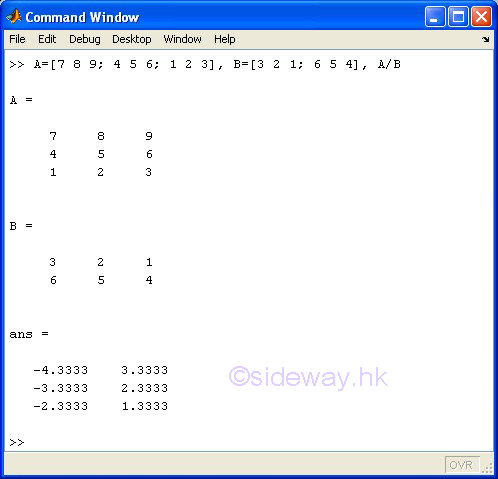• matrix A / scalar S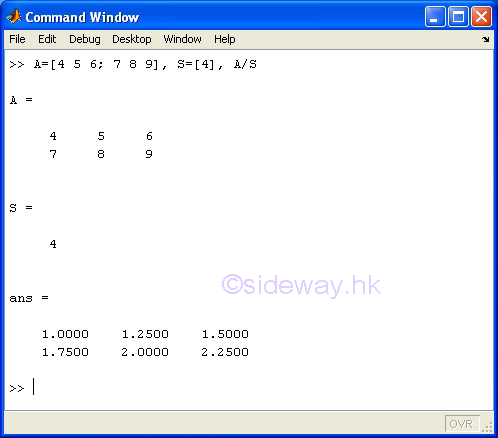#### \ Backslash or Matrix Left Division

The \ backslash or matrix left division operator of the expression A\B means to carry out the linear algebraic product of the inverse of matrix A by matrix B, inv(A)*B, in a general sense of the solution to the equation Ax=B.

Limited by the linear algebraic product of matrix, only a scalar can divide a matrix of any size, unless A is a scalar, otherwise both matrices A and B must have the same number of rows.

#### Examples

• m-by-n matrix A \ m-by-o matrix B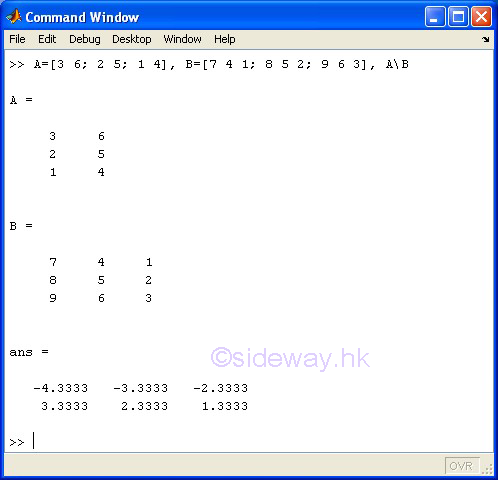• scalar S \ matrix A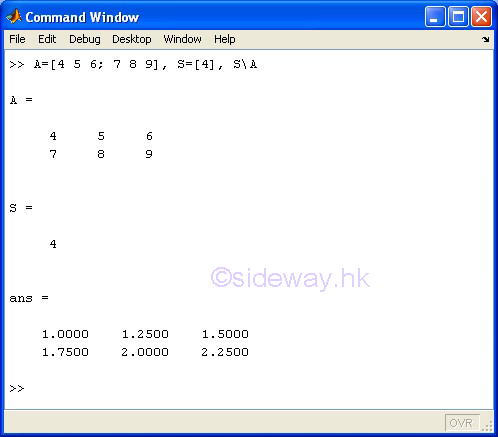#### ^ Matrix Power

The ^ matrix power operator of the expression A^B means to carry out the matrix A to the power of matrix B. However, one of the matrices A and B must be a scalar and the other matrix must be a square matrix.

If B is a scalar of a positive integer and A is a matrix, the matrix power means repeated matrix multiplication of matrix A by itself of the value of scalar B times. If B is scalar of a negative integer, the matrix power means repeated matrix multiplication of the inverse of matrix A by itself to the absolute value of scalar B times. If B is a scalar not equal to an integer and A is a matrix, the matrix power means the calculation involves eigenvalues and eigenvectors, such that if [V,D]=eig(A), then A^B=V*D.^B/V.

If A is a scalar and B is a matrix, the matrix power also means the calculation involves eigenvalues and eigenvectors, such that if [V,D]=eig(A), then A^B=V*D.^B/V.

#### Examples

• square matrix A ^ scalar S of positive integer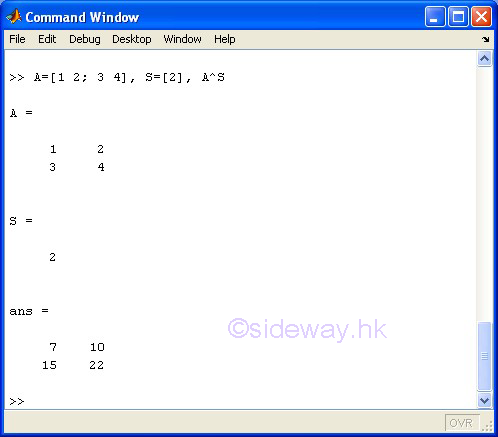• square matrix A ^ scalar S of negative integer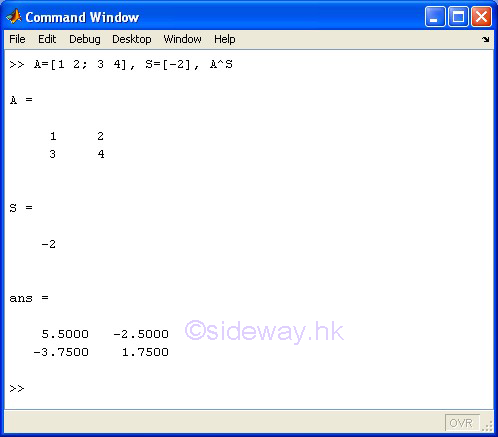• square matrix A ^ scalar S of non-integer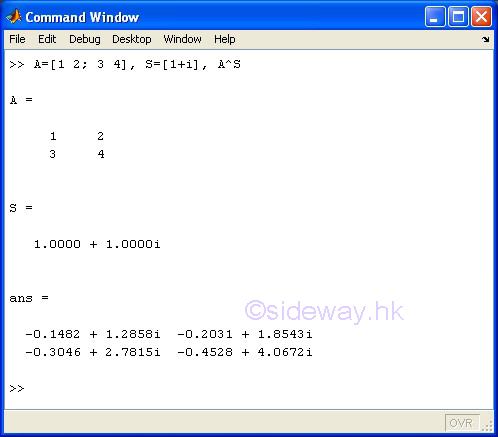• scalar S ^ square matrix A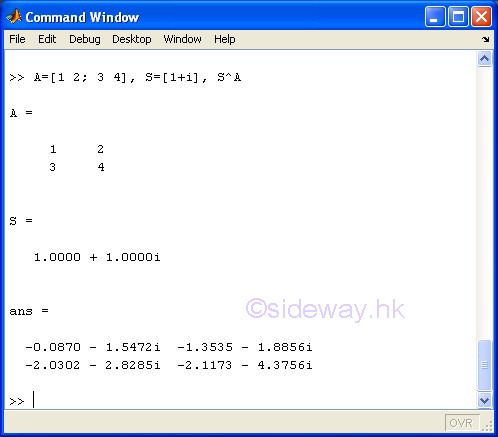#### ' Matrix Transpose or Complex Conjugate Transpose

The ' matrix transpose or complex conjugate transpose operator of the expression A' means to carry out the linear algebraic transpose of matrix A. If matrix A is a complex matrix, the matrix transpose operator means to carry out the complex conjugate transpose.

#### Examples

• m-by-n matrix A'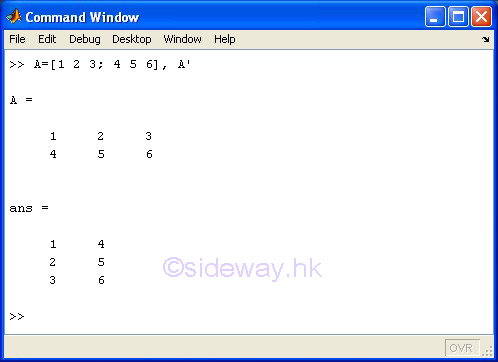• m-by-n complex matrix A'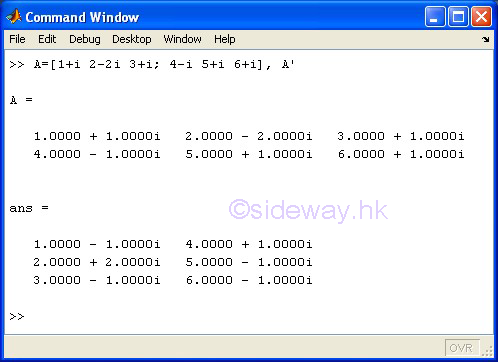### Array Arithmetic Operations

The + addition operator of the expression A+B means adds B to A. Since only a scalar can be added to a matrix of any size, unless either A or B is a scalar, A and B must have the same size.

#### Examples

• m-by-n-by-o array A + m-by-n-by-o array B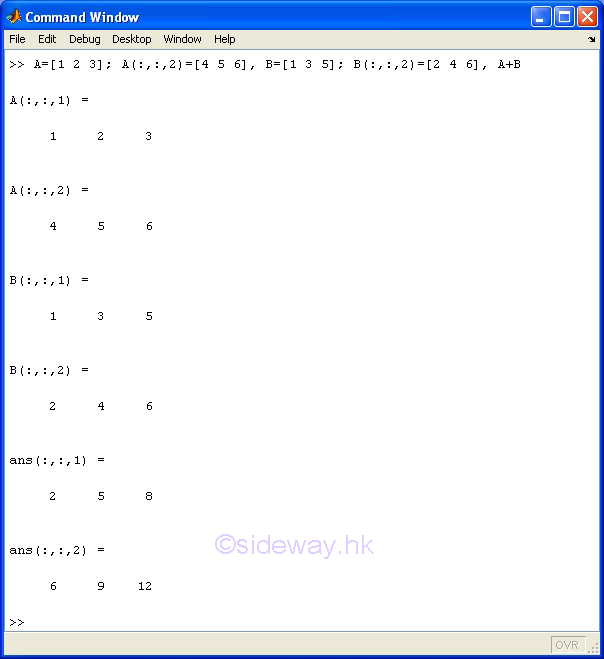• scalar S + m-by-n array A + scalar S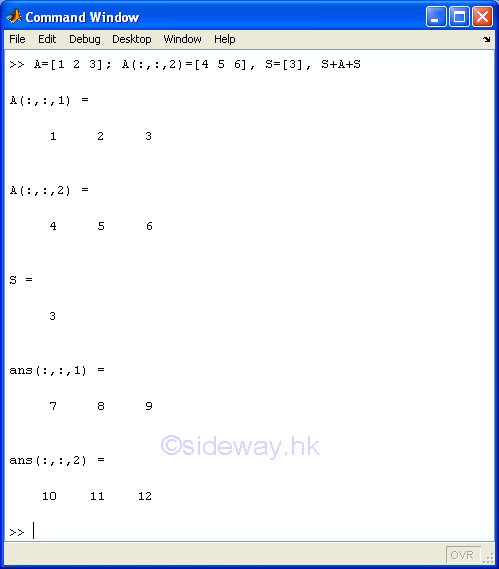#### + Unary Plus

The + unary plus operator of the expression +A means returns A.

#### Examples

• +array A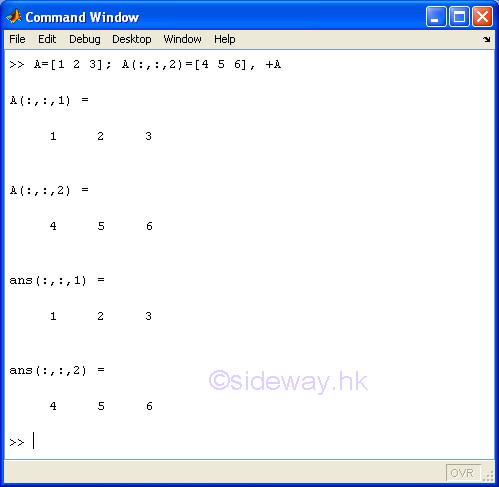#### - Subtraction

The - subtraction operator of the expression A-B means subtracts B from A. Since only a scalar can be subtracted by or subtracted from a matrix of any size, unless either A or B is a scalar, A and B must have the same size.

#### Examples

• m-by-n-by-o array A - m-by-n-by-o array B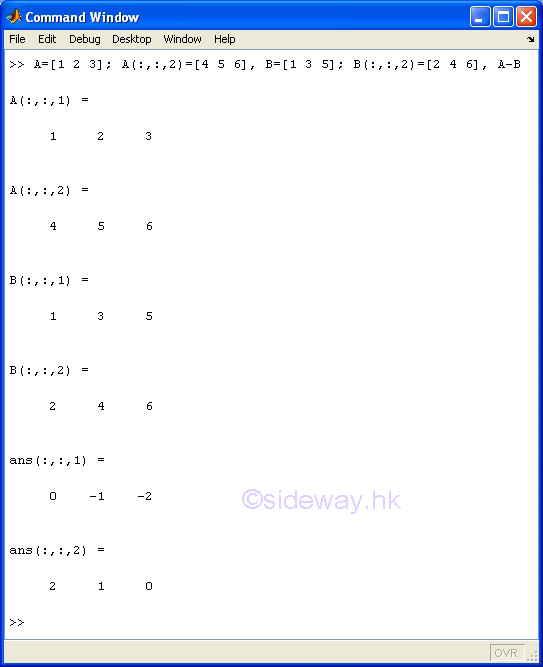• scalar S - m-by-n-by-o array A - scalar S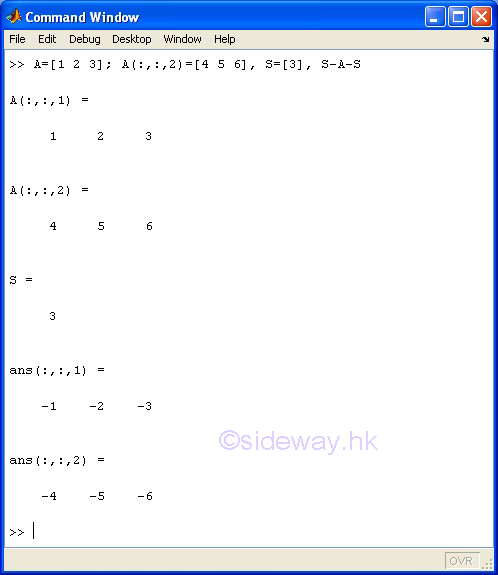#### - Unary Minus

The - unary minus operator of the expression -A means returns and negates the elements of A.

#### Examples

• -array A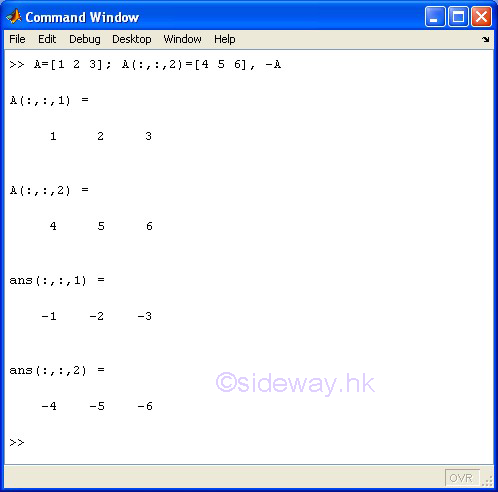#### .* Array Multiplication

The .* array multiplication operator of the expression A.*B means to carry out the element by element product of array A by array B.

Since only a scalar can be multiplicated by or multiplicated to a matrix of any size, unless either A or B is a scalar, otherwise the size of arrays A and B must be equal. Arrays with two or more dimensions are supported.

#### Examples

• m-by-n-by-o array A .* m-by-n-by-o array B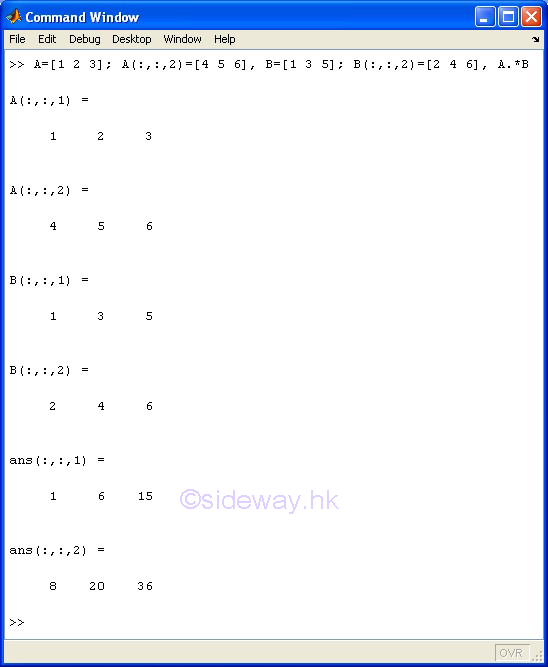• scalar S .* m-by-n-by-o array A .* scalar S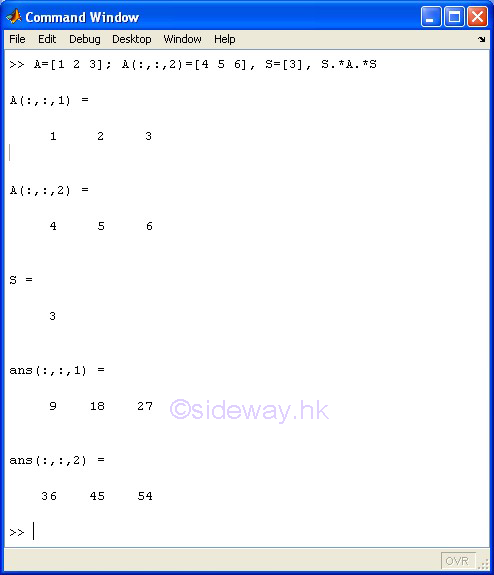#### ./ Array Right Division

The ./ array right division operator of the expression A./B means to carry out the element by element division of array A by array B.

Since only a scalar can be divided by or divide an array of any size, unless either A or B is a scalar, otherwise the size of arrays A and B must be equal. Arrays with two or more dimensions are supported.

#### Examples

• m-by-n-by-o array A ./ m-by-n-by-o array B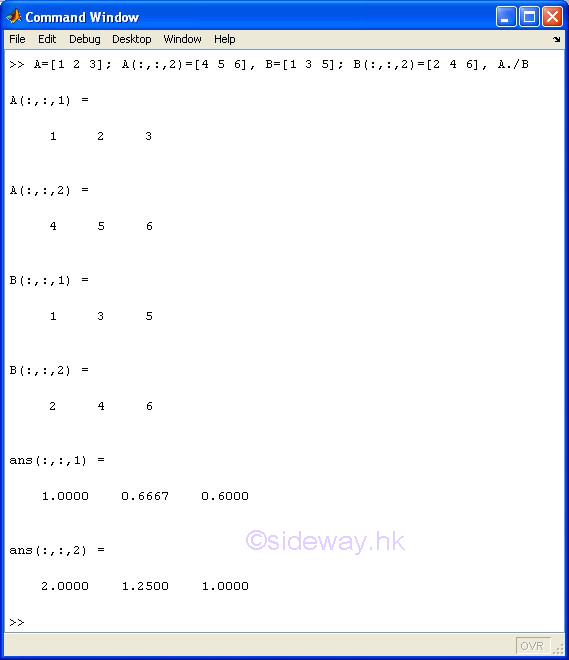• scalar S ./ m-by-n-by-o array A ./ scalar S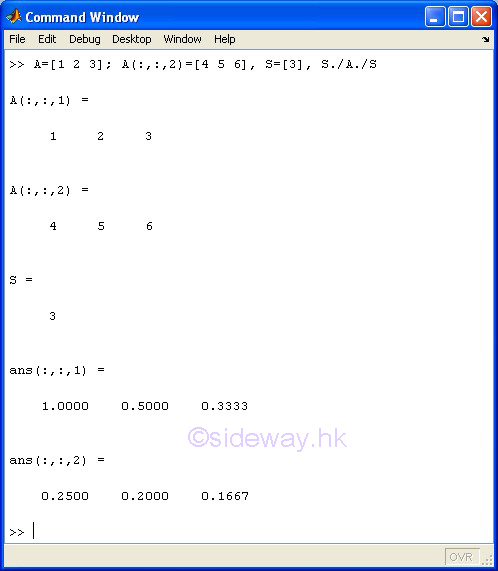#### .\ Array Left Division

The .\ array left division operator of the expression A.\B means to carry out the element by element division of array B by array A.

Since only a scalar can be divided by or divide an array of any size, unless either A or B is a scalar, otherwise the size of arrays A and B must be equal. Arrays with two or more dimensions are supported.

#### Examples

• m-by-n-by-o array A .\ m-by-n-by-o array B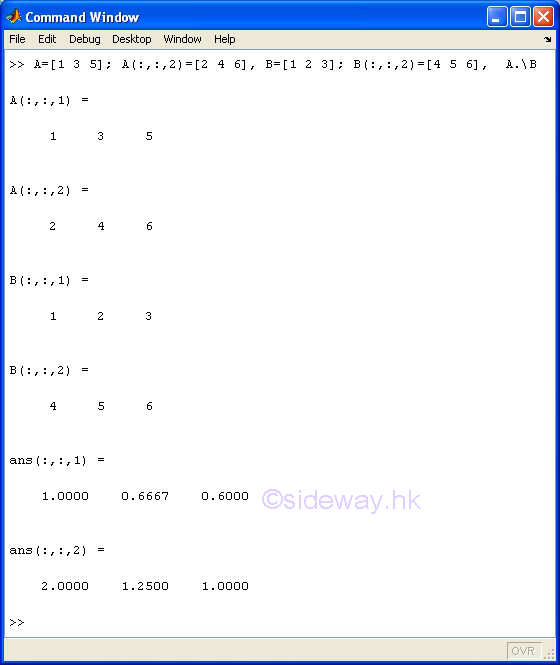• scalar S .\ m-by-n-by-o array A .\ scalar S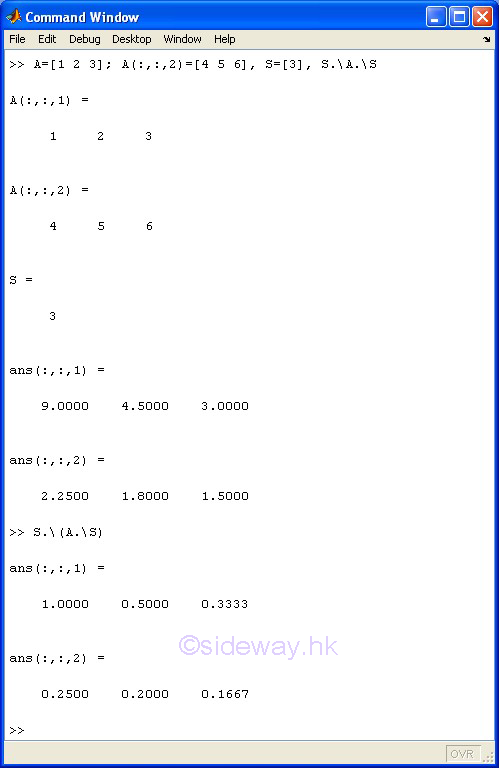#### .^ Array Power

The .^ array power operator of the expression A.^B means to carry out the element by element calculation of the element of array A to the corresponding element of array B.

Since only a scalar can be carried out the element by element operation to an array of any size, unless either A or B is a scalar, otherwise the size of arrays A and B must be equal. Arrays with two or more dimensions are supported.

#### Examples

• m-by-n-by-o array A .^ m-by-n-by-o array B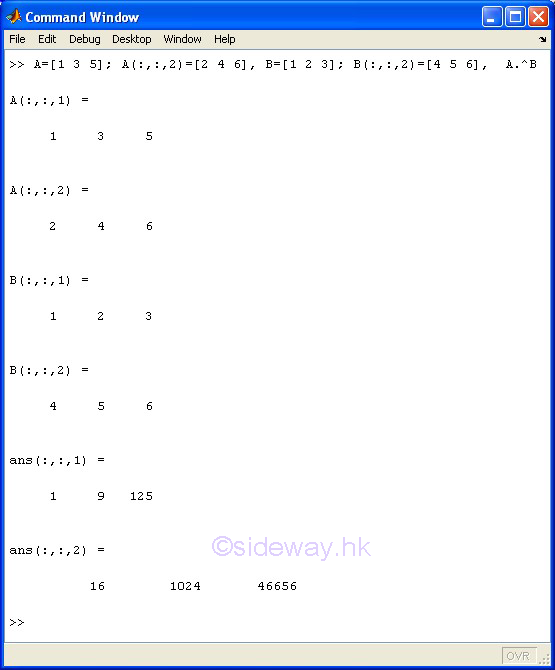• scalar S .^ m-by-n-by-o array A .^ scalar S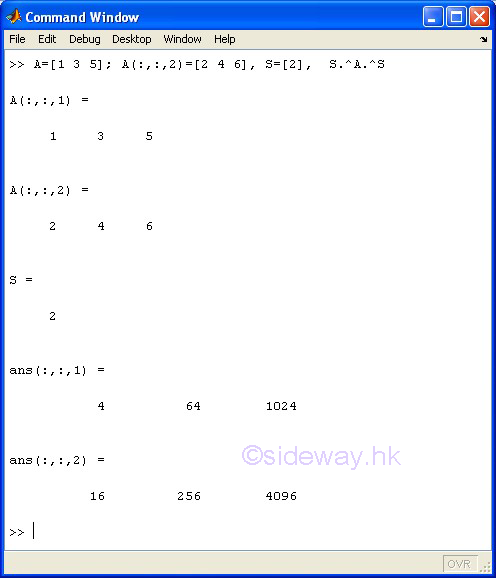#### .' Array Transpose

The .' array transpose of the expression A.' means to carry out the two dimensional array transpose of array A for both real and complex array and no complex conjugation is involved. Only one or two dimensional arrays are supported

#### Examples

• m-by-n array A.'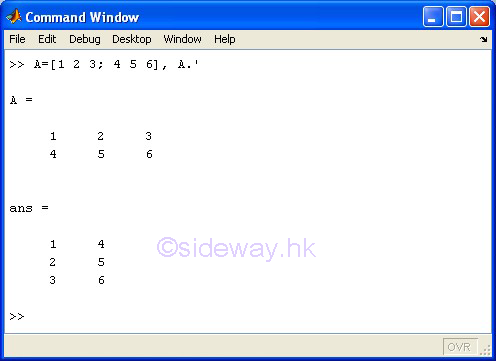• m-by-n complex array A.'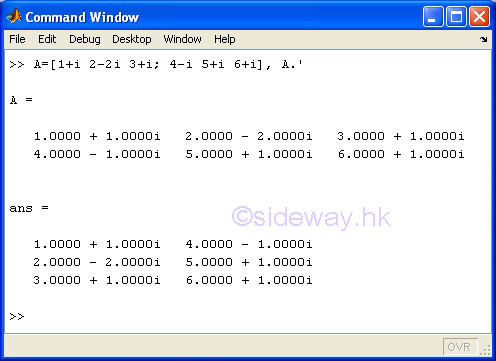### Arithmetic Operation Functions

The function of an arithmetic operator also have its corresponding function call.

Operation Description Function Form
+A Unary Plus uplus(A)
A-B Binary Subtraction minus(A, B)
-A Unary Minus uminus(A)
A*B Matrix
Multiplication
mtimes(A, B)
A/B Forward Slash or Matrix Right Division mrdivide(A, B)
A\B Backslash or Matrix Left Division mldivide(A, B)
A^B Matrix Power mpower(A, B)
A' Matrix Transpose or
Complex Conjugate Transpose
ctranspose(A)
A.*B Array
Multiplication
times(A, B)
A./B Array
Right Division
rdivide(A, B)
A.\B Array
Left Division
ldivide(A, B)
A.^B Array Power power(A, B)
A.' Array Transpose transpose(A)

### Arithmetic Expressions

An arithmetic expression is an expression used to represents a numeric value. Arithmetic expressions are built up from numbers, arithmetic operators and parenthese,  etc. following some predefined rules and operator precedence.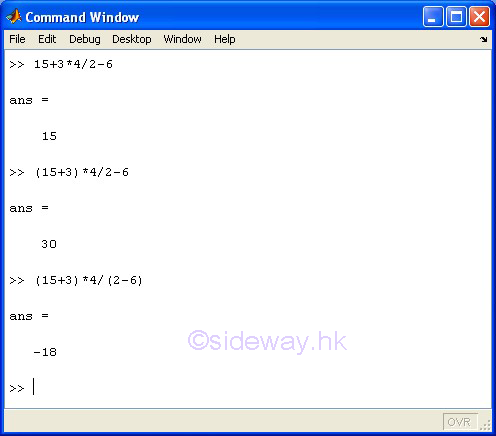### Operator Precedence

An expression is usually formed from a combination of arithmetic, relational, and logical operators. Arithmetic operators are the most common operators used for performing arithmetic calculation on numbers. MatLab always evaluates expressions from left to right, however the order of operators to be evaluated by MatLab within an expression are determined by the precedence levels of operators. The following operator with a higher precedence level will override the rule of from left to right evaluation. While the operator will be evaluated immediately if the following operator has the same or lower precedence level. The precedence rules for MatLab operators are

Precedence Level Operators
1 Parentheses ()
2 Transpose .' ; Power .^ ; Complex conjugate transpose ' ; Matrix power ^
3 Unary plus + ; Unary minus - ; Logical negation ~
4 Multiplication .* ; Right division ./ ; Left division .\ ; Matrix multiplication * ; Matix right division / ; Matrix left division
5 Addition + ; Subtraction -
6 Colon operator :
7 Less than < ; less than or equal to <= ; greater than > ; greater than or equal to >= ; equal to == ; not equal to ~=
8 Element-wise AND &
9 Element-wise OR |
10 Short-circuit AND &&
11 Short-circuit OR ||

Since parentheses have the highest precedence level, parentheses are used to override the default precedence of all other operations.

ID: 140400003 Last Updated: 4/8/2014 Revision: 0Home 5

Management

HBR 3

Information

Recreation

Culture

Chinese 1097

English 339

Computer

Hardware 249

Software

Application 213

Latex 52

Manim 205

KB 1

Numeric 19

Programming

Web 289

Unicode 504

HTML 66

CSS 65

SVG 46

ASP.NET 270

OS 429

Python 72

Knowledge

Mathematics

Algebra 84

Geometry 34

Calculus 67

Engineering

Mechanical

Rigid Bodies

Statics 92

Dynamics 37

Control

Natural Sciences

Electric 27